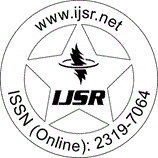International Journal of Science and Research (IJSR)
Call for Papers | Fully Refereed | Open Access | Double Blind Peer Reviewed

Research Paper | Mathematics | Sudan | Volume 5 Issue 3, March 2016

# Estimates of an Eigen Value of Some Isoperimetric Inequalities

Osman Abdallah A. OS | Mohammed Nour A. Rabih | Muntasir Suhail D. S

Abstract: In this paper we give an overview of results about two types of an isoperimetric Inequalities on its eigenvalues and the eigenvalues of the laplacian. We estimate the isoperimetric type constant (M_m) of 2-dimentional Riemannian manifold M_m. if D be the diameter of compact Riemannian manifold M, (n) is the volume of the unit n-dimensional sphere, and i (M) be an injectivity radius of M, we prove that Vol (M) /D> ( (2) ^3) / (54 (3) ^2) i (M) ^2.

Keywords: Riemannian manifold, eigenvalues, compact Riemannian manifold, Laplacian

Edition: Volume 5 Issue 3, March 2016,

Pages: 530 - 535